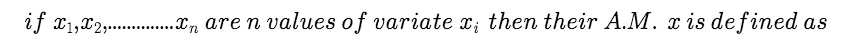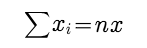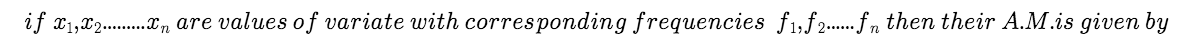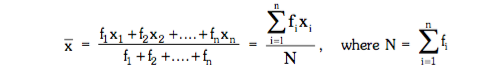# STATISTICS - MEAN, MEDIAN, MODE- Concept with Example

STATISTICS

An average value of a distribution is the value of variable which is representative of the entire distribution, this representative value are called the measures of central tendency.
Generally the following five measures of central tendency.
(a) Mathematical average

(i) Arithmetic mean (ii) Geometric mean

(b) Positional average

(i) Median (ii) Mode

ARITHMETIC MEAN :

1. For ungrouped dist :2.For ungrouped and grouped freq. dist.Post By : Nikita Shah 21 Apr, 2019 2540 views Maths